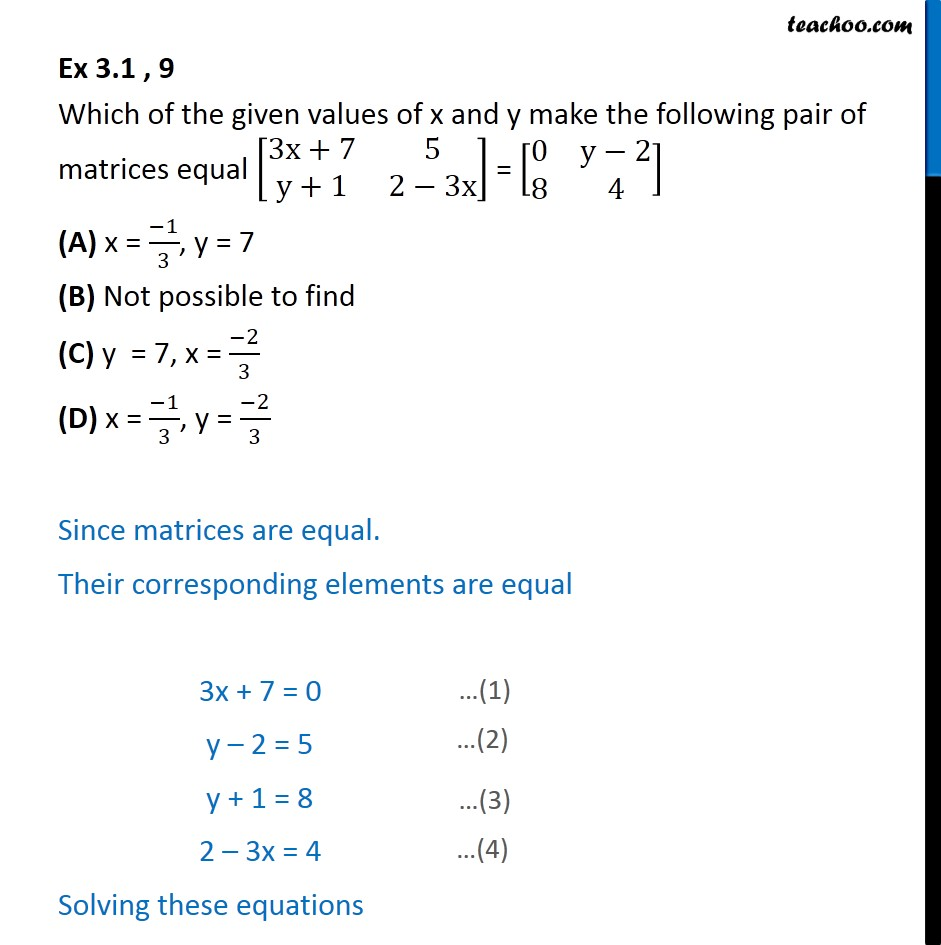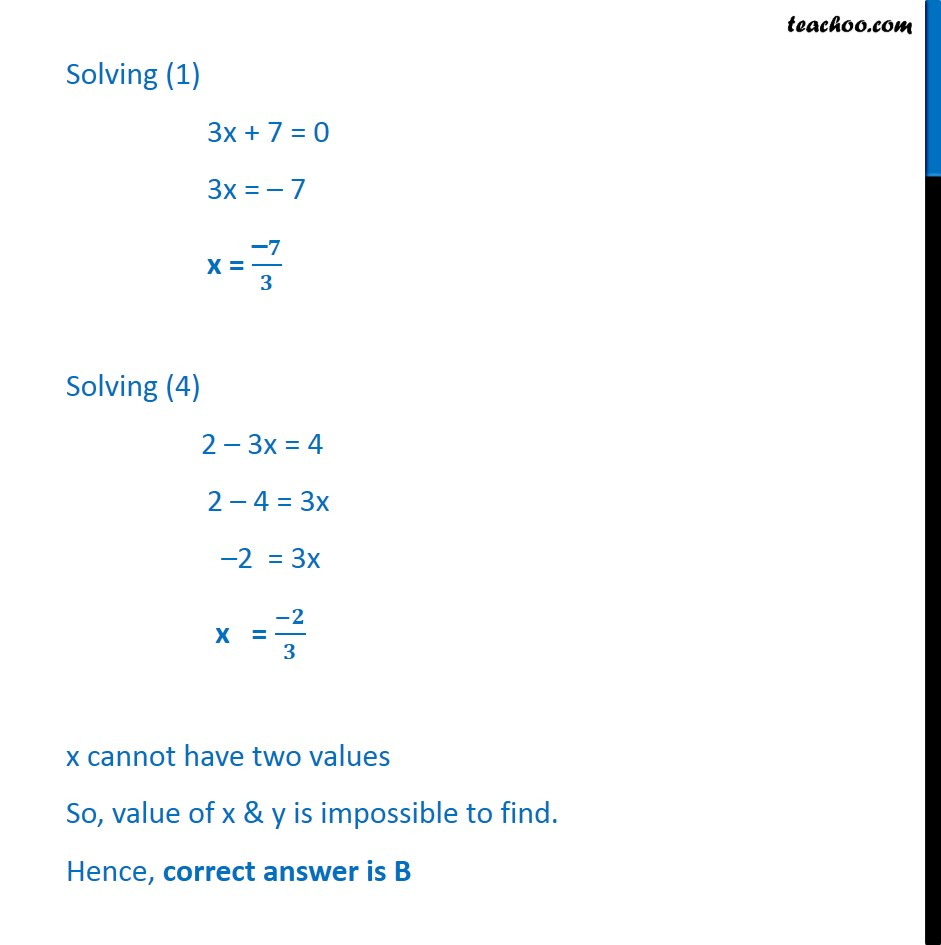1. Chapter 3 Class 12 Matrices
2. Concept wise
3. Equal matrices

Transcript

Ex 3.1 , 9 Which of the given values of x and y make the following pair of matrices equal [ 8(3x+7&5@y+1&2 3x)] = [ 8(0&y 2@8&4)] (A) x = ( 1)/3, y = 7 (B) Not possible to find (C) y = 7, x = ( 2)/3 (D) x = ( 1)/3, y = ( 2)/3 Since matrices are equal. Their corresponding elements are equal 3x + 7 = 0 y 2 = 5 y + 1 = 8 2 3x = 4 Solving these equations Solving (1) 3x + 7 = 0 3x = 7 x = (" " )/ Solving (4) 2 3x = 4 2 4 = 3x 2 = 3x x = ( )/ x cannot have two values So, value of x & y is impossible to find. Hence, correct answer is B

Equal matrices